Home
Hostname: page-component-6c8bd87754-sbrr8 Total loading time: 2.303 Render date: 2022-01-18T10:13:26.737Z Has data issue: true Feature Flags: { "shouldUseShareProductTool": true, "shouldUseHypothesis": true, "isUnsiloEnabled": true, "metricsAbstractViews": false, "figures": true, "newCiteModal": false, "newCitedByModal": true, "newEcommerce": true, "newUsageEvents": true }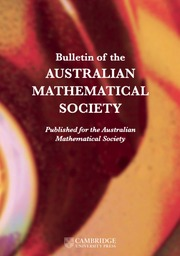Bulletin of the Australian Mathematical Society

CLASSIFICATION OF TETRAVALENT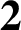$\textbf{2}$-TRANSITIVE NONNORMAL CAYLEY GRAPHS OF FINITE SIMPLE GROUPS

Published online by Cambridge University Press:  11 January 2021

Abstract

A graph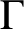$\Gamma$ is called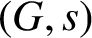$(G, s)$ -arc-transitive if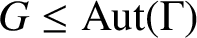$G \le \text{Aut} (\Gamma )$ is transitive on the set of vertices of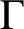$\Gamma$ and the set of s-arcs of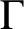$\Gamma$ , where for an integer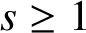$s \ge 1$ an s-arc of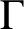$\Gamma$ is a sequence of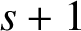$s+1$ vertices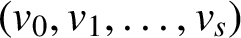$(v_0,v_1,\ldots ,v_s)$ of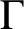$\Gamma$ such that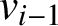$v_{i-1}$ and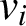$v_i$ are adjacent for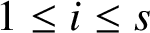$1 \le i \le s$ and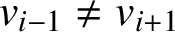$v_{i-1}\ne v_{i+1}$ for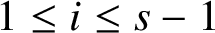$1 \le i \le s-1$ . A graph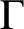$\Gamma$ is called 2-transitive if it is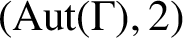$(\text{Aut} (\Gamma ), 2)$ -arc-transitive but not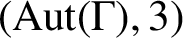$(\text{Aut} (\Gamma ), 3)$ -arc-transitive. A Cayley graph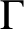$\Gamma$ of a group G is called normal if G is normal in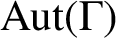$\text{Aut} (\Gamma )$ and nonnormal otherwise. Fang et al. [‘On edge transitive Cayley graphs of valency four’, European J. Combin. 25 (2004), 1103–1116] proved that if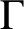$\Gamma$ is a tetravalent 2-transitive Cayley graph of a finite simple group G, then either$\Gamma$ is normal or G is one of the groups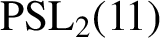$\text{PSL}_2(11)$ ,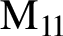${\rm M} _{11}$ ,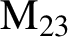$\text{M} _{23}$ and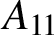$A_{11}$ . However, it was unknown whether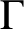$\Gamma$ is normal when G is one of these four groups. We answer this question by proving that among these four groups only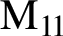$\text{M} _{11}$ produces connected tetravalent 2-transitive nonnormal Cayley graphs. We prove further that there are exactly two such graphs which are nonisomorphic and both are determined in the paper. As a consequence, the automorphism group of any connected tetravalent 2-transitive Cayley graph of any finite simple group is determined.

MSC classification

Type
Research Article
Information
Bulletin of the Australian Mathematical Society , October 2021 , pp. 263 - 271
© 2020 Australian Mathematical Publishing Association Inc.

Access options

Get access to the full version of this content by using one of the access options below. (Log in options will check for institutional or personal access. Content may require purchase if you do not have access.)

Footnotes

X. G. Fang and J. Wang were supported by the National Natural Science Foundation of China (Grant No. 11931005). S. Zhou was supported by the Research Grant Support Scheme of The University of Melbourne.

References

Conway, J. H., Curtis, R. T., Norton, S. P., Parker, R. A. and Wilson, R. A., Atlas of Finite Groups (Clarendon Press, Oxford, 1985).Google Scholar
Fang, X. G., Li, C. H. and Xu, M. Y., ‘On edge transitive Cayley graphs of valency four’, European J. Combin. 25 (2004), 11031116.CrossRefGoogle Scholar
Fang, X. G. and Praeger, C. E., ‘Finite two-arc transitive graphs admitting a Suzuki simple group’, Comm. Algebra 27 (1999), 37273754.CrossRefGoogle Scholar
Fang, X. G., Praeger, C. E. and Wang, J., ‘On the automorphism group of Cayley graphs of finite simple groups’, J. Lond. Math. Soc. 66(2) (2002), 563578.CrossRefGoogle Scholar
Godsil, C. D., ‘On the full automorphism group of a graph’, Combinatorica 1 (1981), 243256.CrossRefGoogle Scholar
Guo, S. T., Feng, Y. Q. and Li, C. H., ‘Edge-primitive tetravalent graphs’, J. Combin. Theory Ser. B 112 (2015), 124137.CrossRefGoogle Scholar
Guralnick, R. M., ‘Subgroups of prime power index in a simple group’, J. Algebra 81 (1983), 304311.CrossRefGoogle Scholar
Lorimer, P., ‘Vertex-transitive graphs: symmetric graphs of prime valency’, J. Graph Theory 8 (1984), 5568.CrossRefGoogle Scholar
Potočnik, P., ‘A list of 4-valent 2-arc transitive graphs and finite faithful amalgams of index$\left(4,2\right)$ ’, European J. Combin. 30 (2009), 1323–1136.CrossRefGoogle Scholar
Sabiddusi, G. O., ‘Vertex-transitive graphs’, Monatsh. Math. 68 (1964), 426438.CrossRefGoogle Scholar
The GAP Group, GAP – Reference Manual, Release 4.7.2, 2013, http://www.gap-system.org.Google Scholar
Xu, M. Y., ‘Automorphism groups and isomorphisms of Cayley graphs’, Discrete Math. 182 (1998), 309319.CrossRefGoogle Scholar
Xu, S. J., Fang, X. G., Wang, J. and Xu, M. Y., ‘On cubic$s$ -arc transitive Cayley graphs of finite simple groups’, European J. Combin. 26 (2005), 133143.CrossRefGoogle Scholar
Xu, S. J., Fang, X. G., Wang, J. and Xu, M. Y., ‘$5$ -arc transitive cubic Cayley graphs on finite simple groups’, European J. Combin. 28 (2007), 10231036.CrossRefGoogle Scholar
1
Cited by

Send article to Kindle

Note you can select to send to either the @free.kindle.com or @kindle.com variations. ‘@free.kindle.com’ emails are free but can only be sent to your device when it is connected to wi-fi. ‘@kindle.com’ emails can be delivered even when you are not connected to wi-fi, but note that service fees apply.

Find out more about the Kindle Personal Document Service.

CLASSIFICATION OF TETRAVALENT$\textbf{2}$-TRANSITIVE NONNORMAL CAYLEY GRAPHS OF FINITE SIMPLE GROUPS Available formats × Send article to Dropbox To send this article to your Dropbox account, please select one or more formats and confirm that you agree to abide by our usage policies. If this is the first time you use this feature, you will be asked to authorise Cambridge Core to connect with your <service> account. Find out more about sending content to Dropbox. CLASSIFICATION OF TETRAVALENT$\textbf{2}$ -TRANSITIVE NONNORMAL CAYLEY GRAPHS OF FINITE SIMPLE GROUPS
Available formats
×

To send this article to your Google Drive account, please select one or more formats and confirm that you agree to abide by our usage policies. If this is the first time you use this feature, you will be asked to authorise Cambridge Core to connect with your <service> account. Find out more about sending content to Google Drive.

CLASSIFICATION OF TETRAVALENT$\textbf{2}\$ -TRANSITIVE NONNORMAL CAYLEY GRAPHS OF FINITE SIMPLE GROUPS
Available formats
×
×